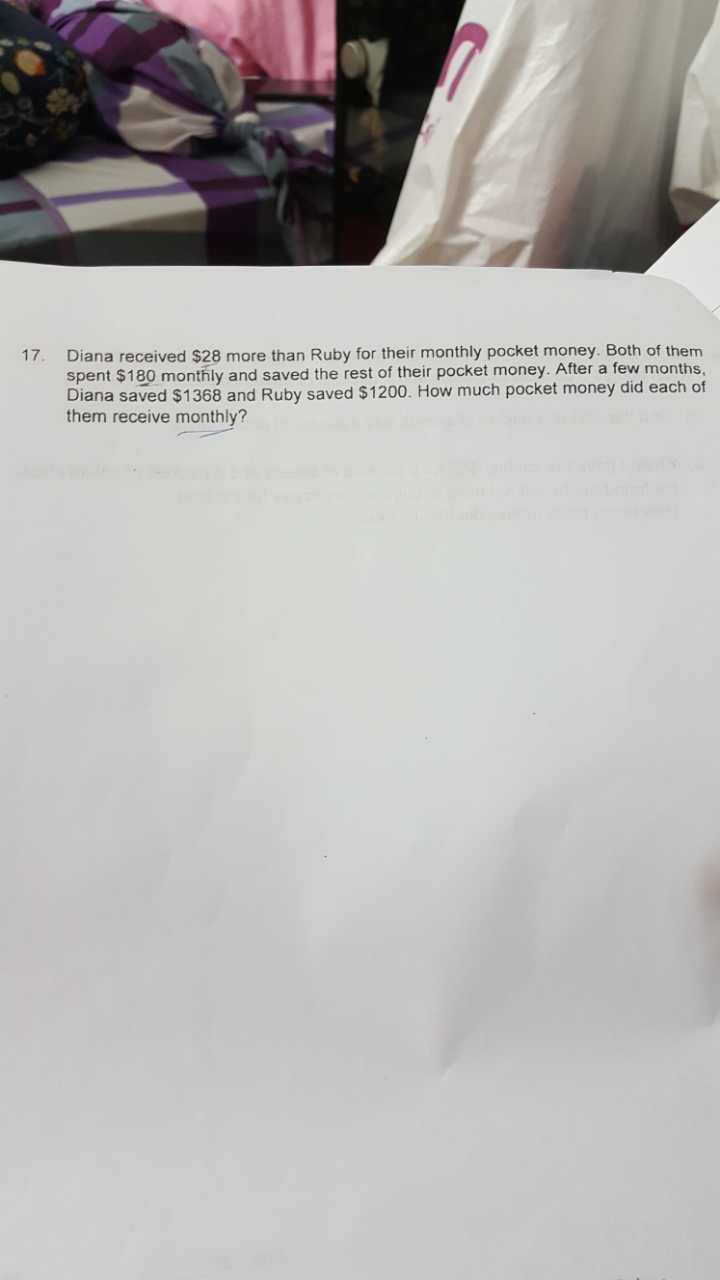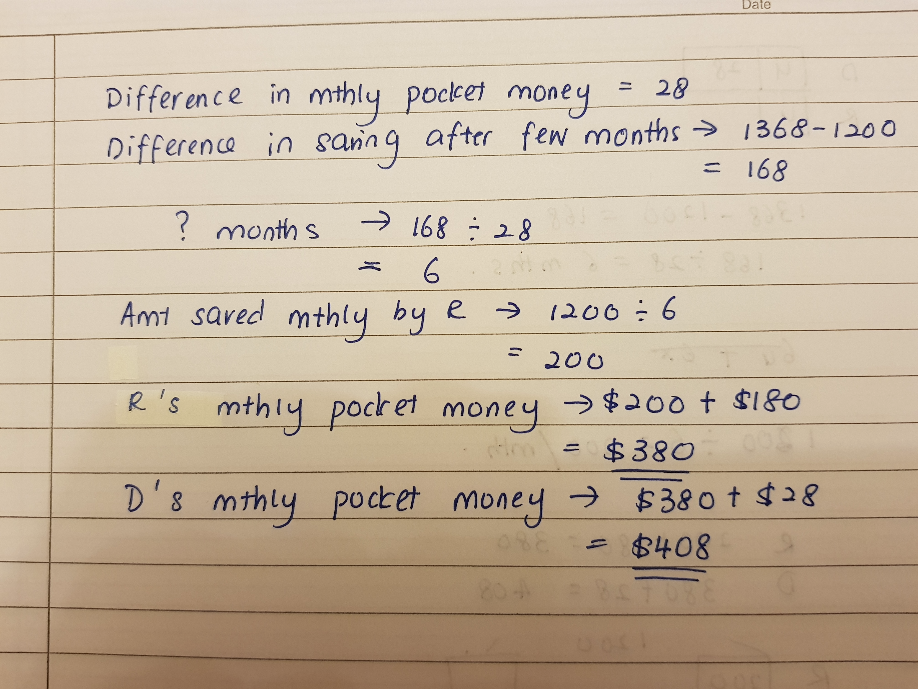# Question4 Answers

# Answer

\$1368 – \$1200 = \$168

\$168 / \$28 = 6

Ruby’s pocket money = (\$1200 / 6) + \$180 = \$380

Diana’s pocket money = \$380 + \$28 = \$408

0 Replies 0 Likes

1368 – 1200 = 168
168/28 = 6 (months)
(1368 + 180 x 6)/6 = 408 (Diana) or (1368/6) + 180 = 408
(1200 + 180 x 6)/6 = 380 (Ruby) or (1200/6) + 180 = 380

Ans : \$408 (Diana) and  \$380 (Ruby).

0 Replies 0 Likes

Difference in amount saved = \$1368 – \$1200 = \$168

Difference in amount received per month = \$28

Number of months to get that difference = \$168 / \$28 = 6

Amount Diana saved per month = \$1368 / 6 = \$228

Amount of pocket money Diana received per month = \$228 + \$180 = \$408

Amount Ruby saved per month = \$1200 / 6 = \$200

Amount of pocket money Ruby received per month = \$2 + \$180 = \$380

1 Reply 1 Like

For diana do we need to add 28 to the value 408 and the answer will be 436 for diana? Please clarify .

1 Reply 0 Likes

No, the total that Diana received is the amount she saved (\$228) and the amount she spent (\$180). This amount is is \$408.

The \$28 is the difference between what she got vs what Ruby got. So that has nothing to do with how much Diana received in absolute terms.

0 Replies 0 Likes0 Replies 1 Like